MathematicsDiscontinuities

`dde23` can solve problems with discontinuities in the history or discontinuities in coefficients of the equations. It provides properties that enable you to supply locations of known discontinuities and a different initial value.

 Discontinuity Property Comments At the initial value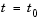`InitialY` Generally the initial value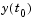is the value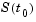returned by the history function, which is to say that the solution is continuous at the initial point. However, if this is not the case, supply a different initial value using the `InitialY` property. In the history, i.e., the solution at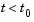, or in the equation coefficients for t sub 0 -->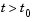`Jumps` Provide the known locations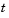of the discontinuities in a vector as the value of the `Jumps` property. State-dependent `Events` `dde23` uses the events function you supply to locate these discontinuities. When `dde23` finds such a discontinuity, restart the integration to continue. Specify the solution structure for the current integration as the history for the new integration. `dde23` extends each element of the solution structure after each restart so that the final structure provides the solution for the whole interval of integration. If the new problem involves a change in the solution, use the `InitialY` property to specify the initial value for the new integration.

Example: Cardiovascular Model

This example solves a cardiovascular model due to J. T. Ottesen . The equations are integrated over the interval [0,1000]. The situation of interest is when the peripheral pressure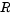is reduced exponentially from its value of 1.05 to 0.84 beginning at= 600.

This is a problem with one delay, a constant history, and three differential equations with fourteen physical parameters. It has a discontinuity in a low order derivative at `t = 600`.

 Note    The demo `ddex2` contains the complete code for this example. To see the code in an editor, click the example name, or type `edit ddex2` at the command line. To run the example type `ddex2` at the command line. See DDE Solver Basic Syntax for more information.

In `ddex2`, the fourteen physical parameters are set as fields in a structure `p` that is shared with nested functions. The function `ddex2de` for evaluating the equations begins with

• ```function dydt = ddex2de(t,y,Z)
if t <= 600
p.R = 1.05;
else
p.R = 0.21 * exp(600-t) + 0.84;
end
.
.
.
```

Solve Using the Jumps Property.   The peripheral pressureis a continuous function of, but it does not have a continuous derivative at `t = 600`. The example uses the `Jumps` property to inform `dde23` about the location of this discontinuity.

• ```opts = ddeset('Jumps',600);
```

After defining the delay `tau` and the constant `history`, the call is

• ```sol = dde23(@ddex2de,tau,history,[0, 1000],opts);
```

The demo `ddex2` plots only the third component, the heart rate, which shows a sharp change at `t = 600`.

Solve by Restarting.   The example could have solved this problem by breaking it into two pieces

• ```sol = dde23(@ddex2de,tau,history,[0, 600]);
sol = dde23(@ddex2de,tau,sol,[600, 1000]);
```

The solution structure `sol` on the interval `[0,600]` serves as history for restarting the integration at `t = 600`. In the second call, `dde23` extends `sol` so that on return the solution is available on the whole interval `[0,1000]`. That is, after this second return,

• ```Sint = deval(sol,[300,900]);
```

evaluates the solution obtained in the first integration at `t = 300`, and the solution obtained in the second integration at `t = 900`.

When discontinuities occur in low order derivatives at points known in advance, it is better to use the `Jumps` property. When you use event functions to locate such discontinuities, you must restart the integration at discontinuities.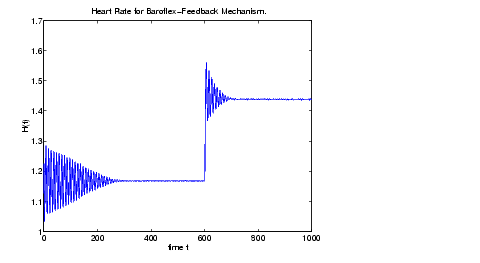Solving DDE Problems Changing DDE Integration Properties© 1994-2005 The MathWorks, Inc.